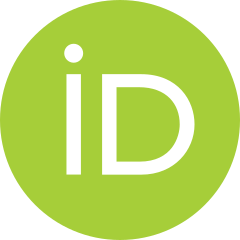# An introduction to harmonic complex numbers and harmonic hybrid Fibonacci numbers: A unified approach

Emel Karaca and Fatih Yılmaz
Notes on Number Theory and Discrete Mathematics
Print ISSN 1310–5132, Online ISSN 2367–8275
Volume 28, 2022, Number 3, Pages 542–557
DOI: 10.7546/nntdm.2022.28.3.542-557

## Details

### Authors and affiliations

Emel KaracaDepartment of Mathematics, Ankara Hacı Bayram Veli University
Ankara, Turkey

Fatih YılmazDepartment of Mathematics, Ankara Hacı Bayram Veli University
Ankara, Turkey

### Abstract

The purpose of this paper is to define and construct new number systems, called the harmonic complex Fibonacci sequences (HCF) and the harmonic hybrid Fibonacci (HHF) sequences. These sequences are defined by inspiring the well-known harmonic and hybrid numbers in literature. We give some fundamental definitions and theorems about these sequences in detail. Moreover, we examine some algebraic properties such as Binet-like-formula, partial sums related to these sequences. Finally, we provide a Maple 13 source code to verify the sequences easily.

### Keywords

• Fibonacci sequence
• Hybrid numbers
• Harmonic numbers

• 11B83
• 11B37
• 05A15

### References

1. Ateş, F., Gök, I., & Ekmekci, N. (2017). Algebraic properties of bi-periodic dual Fibonacci quaternions. Kragujevac Journal of Mathematics, 43, 99–107.
2. Berndt, B. C. (1985). Ramanujan’s Notebooks, Springer, Part I.
3. Berndt, B. C. (1994). Ramanujan’s Notebooks, Springer, Part IV.
4. Catarino, P. (2019). On k-Pell hybrid numbers. Journal of Discrete Mathematical Sciences and Cryptography, 22, 83–89.
5. Coffey, M. W., & Lubbers, N. (2010). On generalized harmonic number sums. Applied Mathematics and Computation, 217, 689–698.
6. Deveci, Ö., Hulku, S., & Shannon, A. G. (2021). On the co-complex k-Fibonacci numbers. Chaos, Solitions & Fractals, 153(2), Article No. 111522.
7. Deveci, Ö., & Shannon, A. G. (2021). The complex-type k-Fibonacci sequences and their applications. Communications in Algebra, 49(3), 1352–1367.
8. Gromov, N. A. (2010). Possible quantum kinematics II. Nonminimal case. Journal of Mathematical Physics, 51, Article No. 083515.
9. Gromov, N. A., & Kuratov, V. V. (2005). All possible Cayley–Klein contractions of quantum orthogonal groups. Physics of Atomic Nuclei, 68, 1689–1699.
10. Gromov, N. A., & Kuratov, V. V. (2006). Possible quantum kinematics. Journal of
Mathematical Physics, 47, Article No. 013502.
11. Halıcı, S. (2012). On Fibonacci quaternions. Advances in Applied Clifford Algebras, 22, 321–327.
12. Hudson, R. (2004). Translation invariant phase space mechanics. Quantum Theory: Reconsideration of Foundations, 2, 301—314.
13. Karaca, E., & Yılmaz, F. (2022). Some characterizations for harmonic complex Fibonacci sequences. In: Yilmaz, F., Queiruga-Dios, A., Santos Sanchez, M.J., Rasteiro, D., Gayoso Mart´ınez, V., & Mart´ın Vaquero, J. (eds) Mathematical Methods for Engineering Applications. ICMASE 2021. Springer Proceedings in Mathematics & Statistics, Vol. 384, 159–165.
14. Kızılateş, C. (2020). A new generalization of Fibonacci hybrid and Lucas hybrid numbers. Chaos, Solitons & Fractals, 130, Article No. 109449.
15. Nurkan, S. K., & Güven, I. A. (2015). Dual Fibonacci quaternions. Advances in Applied Clifford Algebras, 25, 403–414.
16. Özdemir, M. (2018). Introduction to hybrid numbers. Advances in Applied Clifford Algebras, 28, Article No. 11.
17. Study, E. (1903). Geometrie der Dynamen, Cornell Historical Mathematical Monographs at Cornell University Geometrie der Dynamen. Die Zusammensetzung von kräften und verwandte gegenstände der geometrie, Leipzig, B. G. Teubner, 196.
18. Szynal-Liana, A. (2018). The Horadam hybrid numbers. Discussiones Mathematicae – General Algebra and Applications, 38, 91-98.
19. Szynal-Liana, A., & Wloch, I. (2018). On Pell and Pell–Lucas hybrid numbers.
Commentationes Mathematicae, 58(1–2), 11–17.
20. Szynal-Liana, A., & Wloch, I. (2019). Jacobsthal and Jacobsthal–Lucas hybrid numbers. Annales Mathematicae Silesianae, 33, 276–283.

### Manuscript history

• Received: 27 February 2022
• Revised: 4 August 2022
• Accepted: 18 August 2022
• Online First: 20 August 2022

## Cite this paper

Karaca, E., & Yılmaz, F. (2022). An introduction to harmonic complex numbers and harmonic hybrid Fibonacci numbers: A unified approach. Notes on Number Theory and Discrete Mathematics, 28(3), 542-557, DOI: 10.7546/nntdm.2022.28.3.542-557.